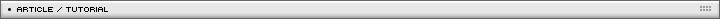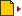Skeletal Animations Page 1/1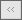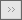[ May 12, 2007 ] Jochen Diehl, aka joeydee An interesting tutorial showing how to create an impressive skeletal-animated human character

This is no true bones animation, just a quick experiment. A set of pre-calculated joints in ten keyframes are interpolated for smooth movement. The flash matrix class is then used to render movie clips instead of lines connecting the joints for a solid appearance.

Use the mouse to rotate around the y axis. Although this prototype is coded in AS3, there is no AS3-only related stuff. The basics should work in AS2 as well, but you will have to rewrite some lines of code like the event listeners and the depth sorting.

How to get an animation array without writing a 3d animation importer:

I used two image sequences of a walking 3d character (rendering joints/bones only suffices), one sequence rendered from the side and the second sequence from front. I used ten frames equally distributed over time (where the 11th would be the same as the 1st), opened them in any imaging program that is able to show the cursor position and wrote down the values of the following joints of each frame:

1 = left shoulder
2 = left ellbow
3 = left hand
4 = left hip
5 = left knee
6 = left heel
7 = left toe

Because I'm lazy, I measured the positions of the left side only and mirrored the values in code later, shifted by five keyframes (the half of the animation).

I used the side view to get the y- (vertical) and z- (horizontal) position, and the front view to get the x- (horizontal) position of every joint in 3d. I took the average of the x values of the head of all frames and substracted it from the x values of all joint positions to center the character in the middle of my coordinate system. The same for the z-values.
All this sounds complicated and labor-intensive. Believe me, it is. If you don't use real live video animation as a source, you should write an importer instead.

The points are stored in my array like this:

```var walk:Array = [
[
],
[
[leftshoulder_frame1_x,...,...],...
],
[...],
[
[lefttoes_frame1_x,...,...],...
]
];

```

An example how to read it:
walk contains an array of all left hip positions, walk contains the 3d position in frame 3, and walk contains the z value of that position.
At this step, walk.length has a value of 8, because we recorded eight points (0-7, see above), walk[n] has a length of 10 (number of keyframes) and walk[n][m] has a length of 3 (x,y,z).

Mirroring the points:

```//mirror
var len=walk.length;
for(pts=0;pts < len;pts++){

n=walk.length;
walk[n]=new Array();
//sizes[n]=sizes[pts];
for(frm in walk[pts]){
walk[n][frm]=new Array();
walk[n][frm]=-walk[pts][(frm+5)%10];
walk[n][frm]=walk[pts][(frm+5)%10];
walk[n][frm]=walk[pts][(frm+5)%10];
}
}
```

This piece of code just mirrors the walk array (and doubles it to 16 entries), where (frm+5)%10 is the shifting value. Change this if you use more or less keyframes than 10.

2) How to connect the joints:

I used a second Array to store connecting information:

```var lines:Array=[
[1,2],[2,3],[4,5],[5,6],[6,7],
[9,10],[10,11],[12,13],[13,14],[14,15],
[16,17],[1,9],[1,4],[9,12],[4,12]
];
```

How to read it: draw a line from joint 1 (left shoulder) to joint 2 (left elbow), another one from elbow to hand and so on

3) How to render a single keyframe:

Ok, many numbers so far, let’s see what they look like. To render a single keyframe, we read the lines array in a loop and use the starting and ending point of each pair to address the walk array of the given frame. To render the front view, use x/y and ignore the z values; to render side view, use z/y and ignore x values.

Pseudo Code:

```For(n in lines)
Startpoint=lines[n]
Endpoint=lines[n]
StartpointCoordinatesArray=walk[Startpoint][myframe];
EndpointCoordinatesArray=walk[Endpoint][myframe];
//render front view
draw a line
from StartpointCoordinatesArray, StartpointCoordinatesArray
to EndpointCoordinatesArray , EndpointCoordinatesArray 
//render side view
draw a line
from StartpointCoordinatesArray, StartpointCoordinatesArray
to EndpointCoordinatesArray , EndpointCoordinatesArray 
```

At this step, I used to loop through the points array as well to draw a circle at every joint position of the given frame. The head wasn’t used in the lines array, so it wouldn’t be drawn when rendering lines only.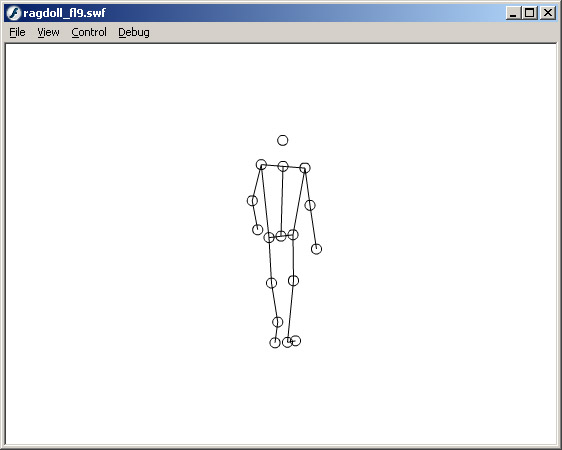4) How to interpolate between keyframes:

For smooth animation, we need to interpolate. We don’t want to render key 3 and key 4 one after another, but calculate 3.1, 3.2, ...,3.9 in between - just like tweening.
For this, we need to calculate:
a) which two frames are necessary to render frame 3.75 for example, and
b) how to “weight” the joints’ positions of both keyframes to get the tweened position.

a) f1: leading frame; f2: following frame (10 is the number of keyframes; the modulo operator is used to loop through the animation, so frame=16 will render frame 6 again)
var f1=int(frame) % 10;
var f2=int(frame+1) % 10;
gives us f1=3 and f2=4 for our example, where frame=3.75

b) fac1: coefficient to weight the following frame’s positions; fac2: coefficient to weight the leading frame’s positions
var fac1=frame-int(frame);
var fac2=1-fac1;
gives us fac1=0.75 and fac2=0.25 for our example.

This means, we have to read the joints’ positions of frame 3, multiplied by 0.25, and add the joints’ positions of frame 4, multiplied by 0.75 to get an interpolated frame at timestep 3.75.

This technique is called “linear interpolation” - I recommend to use google to learn more about different interpolation techniques. There are ways to get more “rounded” movements (bilinear interpolation i.e.), if you need it.

5) How to rotate around the y-axis:

A simple thing, just as you can learn it from any 3d tutorial. Pseudo code:
si=sin(angle)
co=cos(angle)
renderX=pos3dX*co+pos3dZ*si
renderY=pos3dY
renderZ=pos3dZ*co-pos3dX*si

use renderX and renderY to render the joints/lines, renderZ for depth sorting in step 6.

Of course, you can use any 3d transforming operation you want, including multiplying by matrices and even 3d projection with field of view. But I decided to use a simple y rotation only, because I wanted to try some different kind of rendering in the next step:

6) How to render a “solid” model in comic style:

Well, I admit, it’s a bit restricted in design, but it’s relatively fast and very simple in code compared to importing and rendering a real animated 3d model with a cell shader in Flash.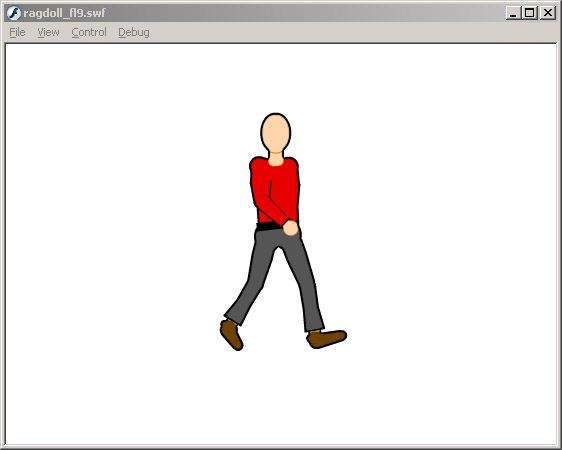The trick is:

- Render a filled circle at each joint.
- Render a fitting rectangle (or trapezoid or any form you need) instead of a line, so the start and the end of the rectangle fit exactly with the underlying circle.
- Use the computed renderZ value (see above) for depth sorting all the shapes
- Finally, use the “glow” filter like shown in Daniel Hunt’s great cell shading tutorial.

I put all pieces into a separate movie clip to which I assigned the glow filter.
All the connecting shapes like arms or legs are basically drawn from left to right and have a width of 100. They are scaled and rotated to fit exactly between two points on the screen then

This is how to use the matrix class to do this job: - put the left edge of MC to p1 (matrix.tx,matrix.ty), - x-scale MC so it’s width fits the distance between p1 and p2 and let the x-axis show towards point 2 (matrix.a, matrix.b) - adjust the y-axis perpendicular to the x axis (matrix.c, matrix.d) - always render y axis upright (the if construct)

```function renderMC(MC,p1,p2){
mat=MC.transform.matrix;
mat.tx=p1/2;
mat.ty=p1/2;
dx=p2-p1;
dy=p2-p1;
d=Math.sqrt(dx*dx+dy*dy);
mat.a=dx/200;
mat.b=dy/200;
mat.c=-dy/d;
mat.d=dx/d;
if(mat.d < 0){
mat.c=-mat.c;
mat.d=-mat.d;
}
MC.transform.matrix=mat;
MC.z=(p1+p2)/2;
}
```

(values are divided by 2 here to fit on a smaller screen.) In the end, I did some tweaking to the scaling and depth sorting for the upper body, and some more tweaking here and there as well.
But after reading this article, you should be able to understand the basic concept, the code in the fla file, and how to adjust it to your needs. I strictly recommend to rewrite the code, using a class structure and comment it well. It was in fact a quick’n’dirty proof of concept only.

If there are AS3 specific questions about depth sorting with the display container model or how to use event listeners, please have a look at the adobe labs references or use google.

7) Ideas where to go from here (it’s your turn now!)

- use time based movement instead of frame based

- try to render a face, maybe by adding further joints, or (much easier) by using a 360-frame-clip where you gotoAndStop() to the frame next to the angle value – see the rotating face of the cell shading tutorial to see what I mean

- the same for the upper body, maybe with a logo on the chest?

- record your own animation sets like “idle”, “jumping”, “running”, “climbing”,... and interpolate between them for smooth transitions

- add field of view and a shadow to walk around in pre-rendered 3d backgrounds as in an adventure game

- use inverse kinematics and rigid body dynamics to create physically based skeletons instead of recorded arrays, like in Thomas Jakobsen great article.

- have fun and post your experiments in the forums